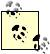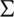# Using Summation Functions

Problem

You're performing calculations that require summations of large amounts of data (for example, for least-squares curve fitting) and would like to use built-in functions that make such summation computations easy.

Solution

Use Excel's built-in summation functions such as SUM, SUMSQ, SUMPRODUCT, and SUMX2MY2.

Discussion

Excel includes several built-in functions that make performing summations of large (or small) amounts of data very easy. Perhaps the most common sum function is SUM, which simply adds all values contained in a range of cells. For example, =SUM(B2:B24) adds all the values contained in cells B2 to B24. The range of cells does not have to be contiguous either. For example, =SUM(B2:B24,C2:C24) adds all the values contained in each cell range (you separate cell ranges with commas). You can even include explicit values like this =SUM(B2:B24,1). In this case, the values contained in the range plus the specified value, 1, are added.Most of Excel's functions that take cell ranges or multiple values as arguments allow you to mix cell references and values, using commas as separators.

Table 7-1 contains a list with short descriptions of several of Excel's convenient summation functions.

Table 7-1. Common summation functions

Function

Syntax

Description

SUM

=SUM(n1, n2, n3, ...)

Returns the sum of all given numbers, which may include cell ranges and explicit numbers.

SUMPRODUCT

=SUMPRODUCT(array1, array2, ...)

Adds the products of corresponding values in given cell ranges (called arrays). The ranges must have the same number of elements.

SUMSQ

=SUMSQ(n1, n2, n3, ...)

Returns the sum of the squares of given numbers, which may include cell ranges and explicit numbers.

SUMX2MY2

=SUMX2MY2(array_x, array_y)

Returns the sum of the difference of squares of values; i.e.,(x2 - y2).

SUMX2PY2

=SUMX2PY2(array_x, array_y)

Returns the sum of the sum of squares of values; i.e.,(x2 + y2).

SUMXMY2

=SUMXMY2(array_x, array_y)

Returns the sum of squares of differences of values; i.e.,[Image_Link]/images/U2211.jpg border=0>(x - y)2.

Excel's Insert Function tool makes it easy to browse for built-in functions. For more information, see Recipe 1.10.

Excel also contains a family of functions that allow you to perform conditional sums, and other operations, on data. For example, you can sum all the values in a range of database-type records that meets a specific criterion. These functions are discussed in Chapter 5.Excel Scientific and Engineering Cookbook (Cookbooks (OReilly))
ISBN: 0596008791
EAN: 2147483647
Year: N/A
Pages: 206
Authors: David M Bourg